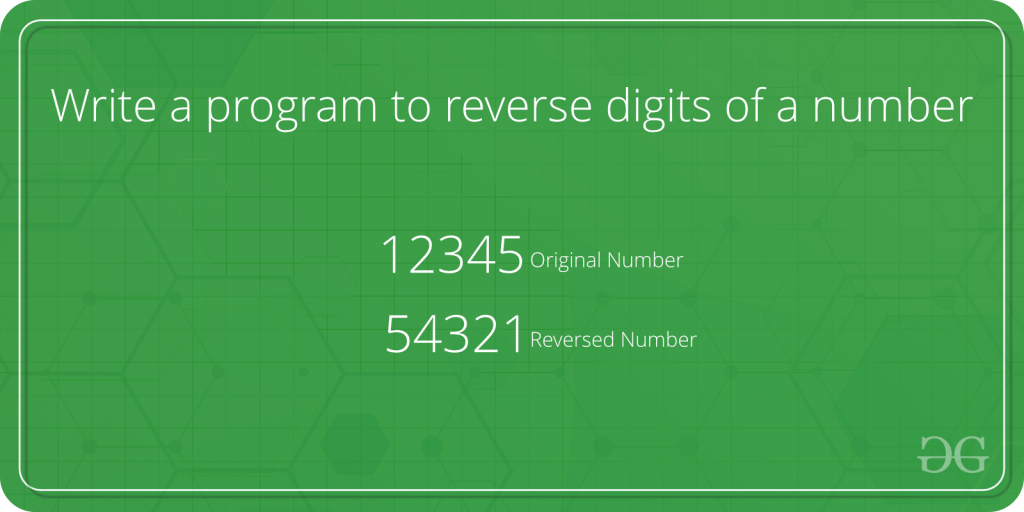# TCS Coding Practice Question | Reverse a Number

Given a number, the task is to reverse this number using Command Line Arguments.

Examples:

```Input: num = 12345
Output: 54321

Input: num = 786
Output: 687
```Approach:

• Since the number is entered as Command line Argument, there is no need for a dedicated input line
• Extract the input number from the command line argument
• This extracted number will be in String type.
• Convert this number into integer type and store it in a variable, say num
• Initialize a variable, say rev_num, to store the reverse of this number with 0
• Now loop through the number num till it becomes 0, i.e. (num > 0)
• In each iteration,
• Multiply rev_num by 10 and add remainder of num to it. This will store the last digit of num in rev_num
• Divide num by 10 to remove the last digit from it.
• After the loop has ended, rev_num has the reverse of num.

Program:

## C

 `// C program to reverse a number ` `// using command line arguments ` ` `  `#include ` `#include      /* atoi */ ` ` `  `// Function to reverse the number ` `int` `reverseNumber(``int` `num) ` `{ ` ` `  `    ``// Variable to store the ` `    ``// resultant reverse number ` `    ``int` `rev_num = 0; ` ` `  `    ``// Traverse through the number digit by digit ` `    ``while` `(num > 0) { ` ` `  `        ``// Append the last digit of num ` `        ``// as the next digit of rev_num ` `        ``rev_num = rev_num * 10 + num % 10; ` ` `  `        ``// Remove the last digit from the num ` `        ``num = num / 10; ` `    ``} ` ` `  `    ``// Return the reversed number ` `    ``return` `rev_num; ` `} ` ` `  `// Driver code ` `int` `main(``int` `argc, ``char``* argv[]) ` `{ ` ` `  `    ``int` `num; ` ` `  `    ``// Check if the length of args array is 1 ` `    ``if` `(argc == 1) ` `        ``printf``(``"No command line arguments found.\n"``); ` `    ``else` `{ ` ` `  `        ``// Get the command line argument and ` `        ``// Convert it from string type to integer type ` `        ``// using function "atoi( argument)" ` `        ``num = ``atoi``(argv); ` ` `  `        ``// Reverse the number and print it ` `        ``printf``(``"%d\n"``, reverseNumber(num)); ` `    ``} ` `    ``return` `0; ` `} `

## Java

 `// Java program to reverse a number ` `// using command line arguments ` ` `  `class` `GFG { ` ` `  `    ``// Function to reverse the number ` `    ``public` `static` `int` `reverseNumber(``int` `num) ` `    ``{ ` ` `  `        ``// Variable to store the ` `        ``// resultant reverse number ` `        ``int` `rev_num = ``0``; ` ` `  `        ``// Traverse through the number digit by digit ` `        ``while` `(num > ``0``) { ` ` `  `            ``// Append the last digit of num ` `            ``// as the next digit of rev_num ` `            ``rev_num = rev_num * ``10` `+ num % ``10``; ` ` `  `            ``// Remove the last digit from the num ` `            ``num = num / ``10``; ` `        ``} ` ` `  `        ``// Return the reversed number ` `        ``return` `rev_num; ` `    ``} ` ` `  `    ``// Driver code ` `    ``public` `static` `void` `main(String[] args) ` `    ``{ ` ` `  `        ``// Check if length of args array is ` `        ``// greater than 0 ` `        ``if` `(args.length > ``0``) { ` ` `  `            ``// Get the command line argument and ` `            ``// Convert it from string type to integer type ` `            ``int` `num = Integer.parseInt(args[``0``]); ` ` `  `            ``// Reverse the number and print it ` `            ``System.out.println(reverseNumber(num)); ` `        ``} ` `        ``else` `            ``System.out.println(``"No command line "` `                               ``+ ``"arguments found."``); ` `    ``} ` `} `

Output:

Attention reader! Don’t stop learning now. Get hold of all the important DSA concepts with the DSA Self Paced Course at a student-friendly price and become industry ready.

My Personal Notes arrow_drop_upCheck out this Author's contributed articles.

If you like GeeksforGeeks and would like to contribute, you can also write an article using contribute.geeksforgeeks.org or mail your article to contribute@geeksforgeeks.org. See your article appearing on the GeeksforGeeks main page and help other Geeks.

Please Improve this article if you find anything incorrect by clicking on the "Improve Article" button below.

Improved By : nidhi_biet

Article Tags :
Practice Tags :

2

Please write to us at contribute@geeksforgeeks.org to report any issue with the above content.